SONAR-INFO-p2018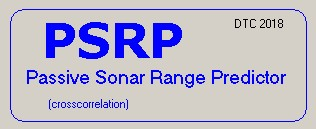1) Preliminary

The PSRP calculator is designed to solve very quickly the problem of determination of the maximum distance of a passive sonar discovery.
PSRP solves the transcendent system in R: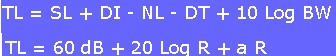after variable insertion:
-SONAR: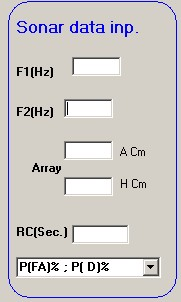-Marine environment: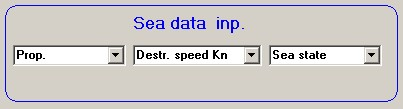2)Detail input data:

SONAR: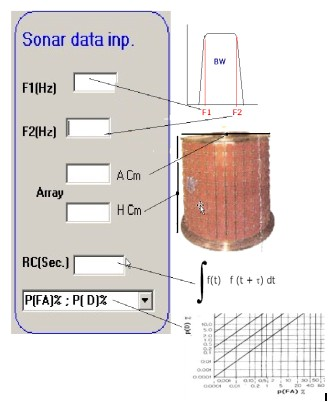Marine environment: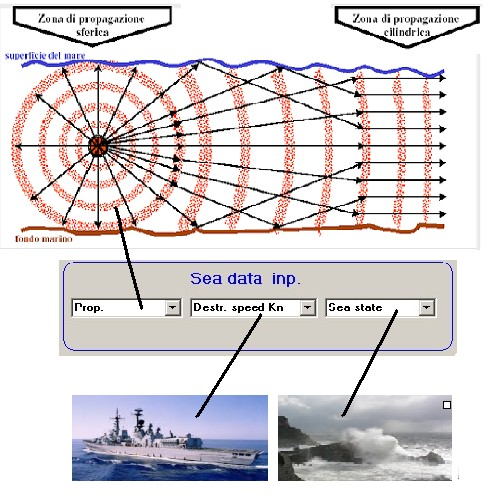3)Intermediate detail results from PSRP:

Data list: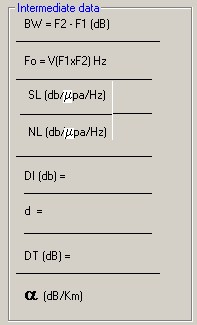4) Detail output data from PSRP

Output data:
Range ( R ) calculated with iterative numerical method :Range ( R ) calculated with graphical method :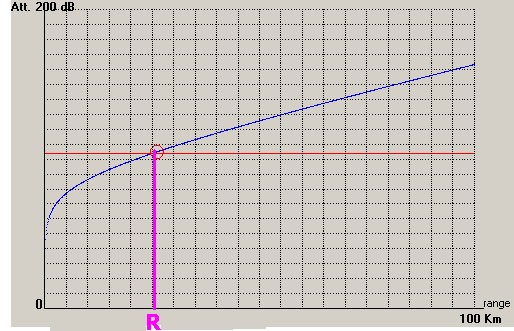5)Example to use PSRP

Input data:

SONAR:
F1 = 8000 Hz
F2 = 12400 Hz
A = 60 cm
H = 90 cm
RC = 1 Sec.
P(FA) = 10%
P(D) = 60%

Marine environment:
Prop. = Sph.
Ds = 15 Kn
SS = 2

we obtain:

-Intermediate data: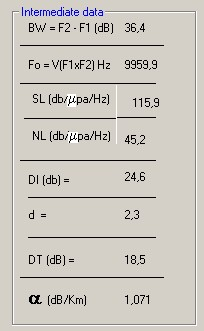-Calculated distance R :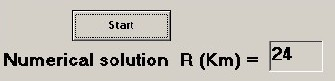Control panel example: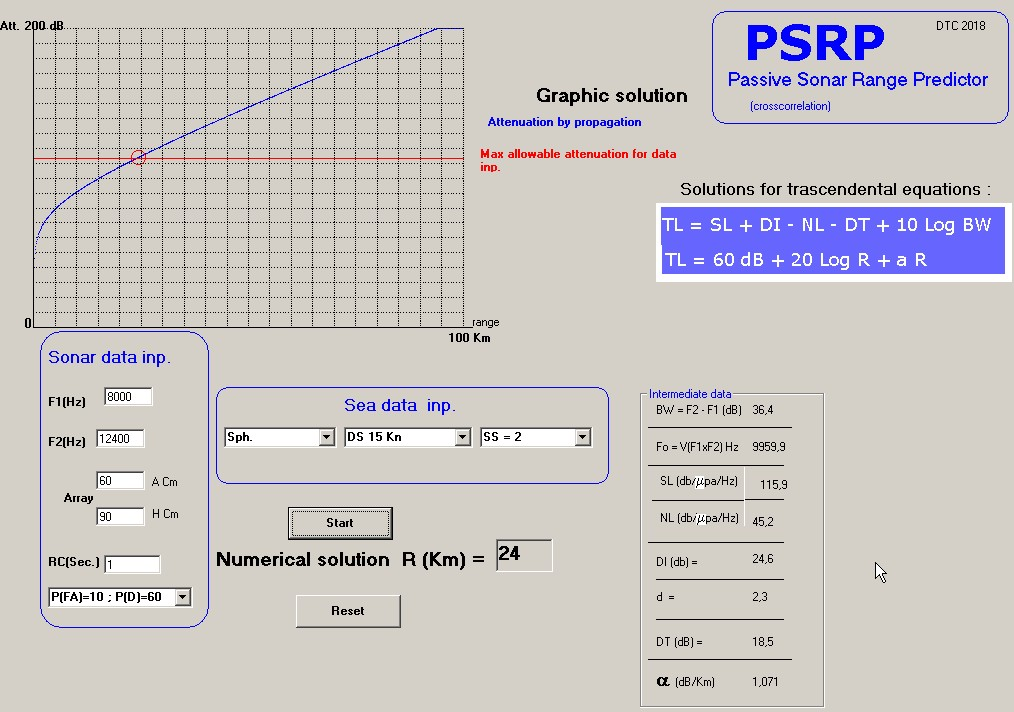PSRP.exe

7)Observations on destroyer's noise levels

The calculated noise levels are related to the second World War destroyers.
At present, new naval units noise levels are not known: that's why we suggest to assume a speed value lower than the one used for the example given.

Home

Stampa immagine grafica o descrizione testuale